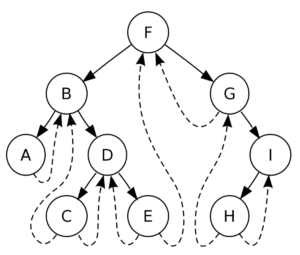# Threaded Binary Tree with Implementation

In this tutorial we are going to see a data structure known as threaded binary tree. Before starting with that we should know what does a binary tree means and after that we can start with the importance of the word threaded in it.

Binary tree is a data structure in computer science where each node has two children represented as left and right children and each node is storing some data.

The above is a basic structure of a binary tree. The left and right pointer points to the left and right node of the current node.

### What does the word threaded mean?When a particular node is at the last depth in a binary tree (also known as leaf node) it does not have a left or right child and generally they are set to NULL. But with threaded binary tree the left and right pointers are set to point the inorder predecessor and inorder successor respectively.

What do we mean by inorder predecessor or inorder successor?

Inorder predecessor is the node coming before the current node in the inorder traversal of a binary tree. Similarly, Inorder successor is the node coming next to the current node in the inorder traversal of a binary tree.

So the order of nodes in inorder traversal will be as :

…   inorder_predecessor -> current_ele -> inorder_successor ….

Now one more question remains is that how will we know that the left and right pointers are pointing to the child or the predecessor and successor?

For solving this a new boolean variable is stored in the structure of a linked list node which is true if the tree is threaded for the current node otherwise it’s false. The new structure will look as below :

### Why do we need threaded binary trees?

The structure of the node of binary tree is already decided and is the same for every node but the space is wasted for the leaf node because they do not store any address.

Generally when we do inorder traversal of a binary tree we either need stack or use recursion which again uses stack at some level i.e. extra memory is required for the inorder traversal. By using these threads we can actually simplify the inorder traversal so that it does not use any space for the inorder traversal. So for memory optimizations threaded binary trees are used.

### Types of Threaded Binary Tree

Based on the pointers threaded in a node there are two types of threaded binary trees

Single Threaded Trees: For every node only one of the pointers is threaded i.e. either the left node is made to point the inorder predecessor OR the right node is made to point to the inorder successor of the tree.

Double Threaded Trees: For every node both of the pointers are threaded i.e. either the left node is made to point the inorder predecessor AND the right node is made to point to the inorder successor of the tree.

### Threaded Binary Search Tree Implementation

We will now see how we can insert and delete elements in a threaded binary search tree. We will use a Double threaded tree for demonstration as Double threaded tree can easily be converted into Single Threaded tree.

Insertion:

Basic insertion will follow the same rules as binary search tree, only additional thing we need to implement is updating the pointers and the value of left and right pointers and the boolean values rightThreaded and leftThreaded.

First of all we need to find the correct place of the new node in the tree using the rules same as we use for binary search tree and thereafter there can be three cases when we are inserting an element in a tree :

• First element
• Left Child
• Right Child

Case 1: First element

While adding the first element there is no meaning of updating the predecessor and successor as they do not exist, so we will just create a new update with left and right pointers as null and update the root of the tree. temp is the new node we created.

Case 2: Left Child

If the tree is not empty then if the value to be inserted (say val) is less than the node value then we will go left otherwise we will go right until we reach a leaf of the tree. The last node we encounter in the process will become the parent of the new node. If val is lesser than the value of parent node it will become its left child.

While writing the inorder of the tree after inserting the node the newly inserted node will come in between the predecessor of parent and parent itself so the predecessor of the new node will become the predecessor of parent and successor of the new node will parent.

If temp is the new node created and parent is the parent of the new node the code will look as below :

Case 3: Right Child

We will follow the same procedure to find the position of the node using the same procedure and if the parent value if less than a new node value, the new node will now become the right child of the parent.

Similar to the Case 2 here the successor of the new node will the successor of the parent and predecessor will become the parent itself.

The final code for insertion will look as below:

Comment down below if you have any queries related to threaded binary tree.

### 1 thought on “Threaded Binary Tree with Implementation”

1.Sir, please share the program of inorder traversal in double threaded binary tree